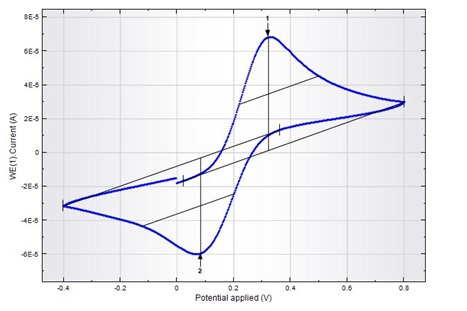# Electrochemical Analyser

### Principle of electrochemical analyser:

Electrochemistry is a branch of chemistry that studies chemical reactions which take place in a solution at the interface of an electron conductor (the electrode: a metal or a semiconductor) and an ionic conductor (the electrolyte). These reactions involve electron transfer between the electrode and the electrolyte or species in solution. Thus electrochemistry deals with interactions between electrical energy and chemical change and vice versa.

### Three electrode setup:

The three-electrode cell setup is the most common electrochemical cell setup used in electrochemical analyser (see Figure). In this case, the current flows between the CE and the WE. The potential difference is controlled between the WE and the CE and measured between the RE (kept at close proximity of the WE) and S. Because the WE is connected with S and WE is kept at pseudo-ground (fixed, stable potential), by controlling the polarization of the CE, the potential difference between RE and WE is controlled all the time. The potential between the WE and CE usually is not measured. This is the voltage applied by the control amplifier and it is limited by the compliance voltage of the instrument. It is adjusted so that the potential difference between the WE and RE will be equal to the potential difference specified by the user. This configuration allows the potential across the electrochemical interface at the WE to be controlled with respect to the RE. To decrease the ohmic losses due to the existence of residual solution between the RE and WE (called the Uncompensated resistance), a so-called Luggin-Haber (or just Luggin) capillary can be used to bring the RE extremity as close as possible to the surface of the WE (see Figure). Since almost no current flows into the reference electrode (typically, the leakage current is a few pA at most), there is little or no voltage drop across the capillary, ensuring that the extremity of the capillary is at a potential very close to the potential of the RE.Application of Electrochemical Analyser:

• Cyclic voltammetry
• Differential pulse voltammetry
• Linear sweep voltammetry
• Square wave voltammetry
• Chrono amperometry
• Chrono potentiometry
•• Figure: Cyclic voltammetric curve of Ferro-Ferricyanide